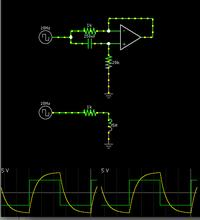# Gyrator simulated inductor design?

Status
Not open for further replies.

#### mr_monster

##### Member level 4
I would like to make a simulated inductor which is tune-able using an opamp implementation of an opamp. I could find a good source for the design process so anything like that would be greatly appreciated. Thanks!

Falstad's animated simulator includes a gyrator (active inductor) in its menu of circuits.

Screenshot:https://tinyurl.com/axjyjqz

You can change values by right-clicking a component and choosing Edit.

I don't know how to make it tunable.

Thanks for the link! However I did not see any formulas or anything on there? I would like to know how to set the inductor parameters using part selection... thanks!

Yes, I believe it works like this...

The upper schematic shows 250 nF & 20k (the resistor to ground). The capacitive time constant (R x C) comes out to .005.

The lower schematic shows 5H & 1k. The inductive time constant (L/R) is .005. This matches the upper example as to math and performance.

So for a given capacitor value in the gyrator, you can tune its effective Henry value by adjusting the resistor to ground.

I would like to make a simulated inductor which is tune-able using an opamp implementation of an opamp. I could find a good source for the design process so anything like that would be greatly appreciated. Thanks!

As you will have noted - the circuit as given in post#2 is an active lossy inductor.
In case you need a lossless inductor you must use a GIC implementation using two opamps.

As you will have noted - the circuit as given in post#2 is an active lossy inductor.
In case you need a lossless inductor you must use a GIC implementation using two opamps.

When you say lossless, you mean without series resistance? What would be a Q of such an inductor if that is even discussable in those terms? What about the Q of a simulated inductor? reactance / resistance?
I can make a tunable version by using a variable resistor instead of resistor going to ground?

When you say lossless, you mean without series resistance? What would be a Q of such an inductor if that is even discussable in those terms? What about the Q of a simulated inductor? reactance / resistance?
I can make a tunable version by using a variable resistor instead of resistor going to ground?

"Lossless" means: Without resistive losses. Thus, under ideal conditions Q approaching infinity.
In reality - no opamp is ideal and, thus, no GIC implementation is ideal leading to a finite Q - however very large (depending on the opamp type and the operating frequency).

I would like to make a simulated inductor which is tune-able using an opamp implementation of an opamp. I could find a good source for the design process so anything like that would be greatly appreciated. Thanks!

Here is another information which may be helpful for you:

There is another single-opamp active inductor with a parallel loss resistor (instead of a series loss resistor as shown in BradtheRads answer).
It is based on the integrator principle. If you are interested - give notice.

Sure I would like to see that!

Sure I would like to see that!

Its quite simple:

Take the classical inverting MILLER integrator (R2, C2) and use another resistor R1 between opamp output and signal input (left node of R2).
Then you get an impedance Xp=(Rp||jwL) with Rp=R1||R2 and L=R1*R2*C2.

Status
Not open for further replies.